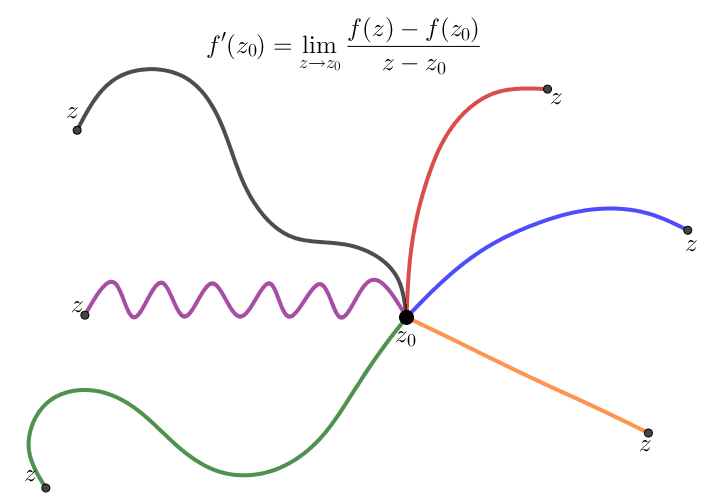# Complex Differentiation

The notion of the complex derivative is the basis of complex function theory. The definition of complex derivative is similar to the derivative of a real function. However, despite a superficial similarity, complex differentiation is a deeply different theory.

A complex function $f(z)$ is differentiable at a point $z_0\in \mathbb C$ if and only if the following limit difference quotient exists

\begin{eqnarray}\label{diff01} f'(z_0) = \lim_{z \rightarrow z_0} \frac{f(z)-f(z_0)}{z-z_0}. \end{eqnarray}

Alternatively, letting $\Delta z = z-z_0$, we can write

\begin{eqnarray}\label{diff02} f'(z_0) = \lim_{\Delta z \rightarrow 0} \frac{f(z_0+\Delta z)-f(z_0)}{\Delta z}. \end{eqnarray}

We often drop the subscript on $z_0$ and introduce the number $\Delta w = f(z+\Delta z)-f(z).$ which denotes the change in the value $w=f(z)$ corresponding to a change $\Delta z$ in the point at which $f$ is evaluated. Then we can write equation (\ref{diff02}) as $\frac{d w}{d z}= \lim_{\Delta z \rightarrow 0}\frac{\Delta w}{\Delta z}.$

Despite the fact that the formula (\ref{diff01}) for a derivative is identical in form to that of the derivative of a real-valued function, a significant point to note is that $f'(z_0)$ follows from a two-dimensional limit. Thus for $f'(z_0)$ to exist, the relevant limit must exist independent of the direction from which $z$ approaches the limit point $z_0$. For a function of one real variable we only have two directions, that is, $x\lt x_0$ and $x\gt x_0$.A remarkable feature of complex differentiation is that the existence of one complex derivative automatically implies the existence of infinitely many! This is in contrast to the case of the function of real variable $g(x)$, in which $g'(x)$ can exist without the existence of $g''(x)$.

## Cauchy-Riemann equations

Now let's see a remarkable consequence of definition (\ref{diff01}). First we will see what happens when we approach $z_0$ along the two simplest directions - horizontal and vertical. If we set $$z= z_0 + h = (x_0+h)+iy_0,\quad h\in \mathbb R,$$ then $z \rightarrow z_0$ along a horizontal line as $h\rightarrow 0.$ If we write $f$ in terms of its real and imaginary components, that is $$f(z) = u(x,y)+iv(x,y),$$ then $$f'(z_0)= \lim_{h \rightarrow 0}\frac{f(z_0+h)-f(z_0)}{h}$$ then

\begin{eqnarray*} f'(z_0)&= & \lim_{h \rightarrow 0} \frac{f(z_0+h)-f(z_0)}{h} = \lim_{h \rightarrow 0} \frac{f(x_0+h + iy_0)-f(x_0+iy_0)}{h} \\ &= & \lim_{h \rightarrow 0} \left[ \frac{u \left( x_0 +h, y_0 \right) - u \left( x_0 , y_0 \right)}{h}\right]+i \lim_{h \rightarrow 0} \left[ \frac{v \left( x_0 +h, y_0 \right) - v \left( x_0 , y_0 \right)}{h}\right] \\ &= & u_x(x_0, y_0)+ i v_x(x_0,y_0) \end{eqnarray*}

where $u_x(x_0, y_0)$ and $v_x(x_0,y_0)$ denote the first-order partial derivatives with respect to $x$ of the function $u$ and $v$, respectively, at $(x_0, y_0)$. If now we set $$z = z_0+ik = x_0 + i(y_0+k), \quad k\in \mathbb R,$$ then $z\rightarrow 0$ along a vertical line as $k\rightarrow 0$. Therefore, we also have

\begin{eqnarray*} f'(z_0)&= & \lim_{k \rightarrow 0} \frac{f(z_0+ik)-f(z_0)}{ik} = \lim_{k \rightarrow 0} \left[ -i \frac{f(x_0 + i(y_0+k))-f(x_0+iy_0)}{k} \right] \\ &= & \lim_{k \rightarrow 0} \left[ \frac{v \left( x_0 , y_0 + k\right) - v \left( x_0 , y_0 \right)}{k}-i \frac{u \left( x_0 , y_0 +k \right) - u \left( x_0 , y_0 \right)}{k}\right] \\ &= & v_y(x_0, y_0)- i u_y(x_0,y_0) \end{eqnarray*}

where the partial derivatives of $u$ and $v$ are, this time, with respect to $y$. By equating the real and imaginary parts of these two formulae for the complex derivative $f'(z_0)$, we notice that the real and imaginary components of $f(z)$ must satisfy a homogeneous linear system of partial differential equations: $$u_x=v_y, \quad u_y=-v_x.$$ These are the Cauchy-Riemann equations named after the famous nineteenth century mathematicians Augustin-Louis Cauchy and Bernhard Riemann, two of the founders of modern complex analysis.

Theorem 1: A complex function $f(z)=u(x,y)+iv(x,y)$ has a complex derivative $f'(z)$ if and only if its real and imaginary part are continuously differentiable and satisfy the Cauchy-Riemann equations \begin{eqnarray*} u_x=v_y, \quad u_y=-v_x \end{eqnarray*} In this case, the complex derivative of $f(z)$ is equal to any of the following expressions: $$f'(z)=u_x+iv_x = v_y - i u_y.$$

Example: Consider the function $f(z)=z^2$, which can be written as $$z^2 = \left(x^2-y^2\right)+ i \left(2xy\right).$$ Its real part $u = x^2-y^2$ and imaginary part $v=2xy$ satisfy the Cauchy-Riemann equations, since $$u_x=2x = v_y, \quad u_y = -2y = -v_x.$$ Theorem 1 implies that $f(z)=z^2$ is differentiable. Its derivative turns out to be

$$f'(z)=u_x+iv_x = v_y - i u_y = 2x + i 2y = 2(x+iy) = 2z.$$

Fortunately, the complex derivative has all of the usual rules that we have learned in real-variable calculus. For example,

$$\frac{d}{dz}z^n = nz^{n-1}, \quad \frac{d}{dz} e^{cz} = ce^{cz}, \quad \frac{d}{dz} \log z = \frac{1}{z},$$

and so on. In this case, the power $n$ can be a real number (or even complex in view of the identity $z^n = e^n \log z$), while $c$ is any complex constant. The exponential formulae for the complex trigonometric and hyperbolic functions implies that they also satisfy the standard rules

\begin{eqnarray*} \frac{d}{dz}\sin z &=& \cos z, \quad \frac{d}{dz} \cos z = -\sin z.\\ \frac{d}{dz}\sinh z &=& \cosh z, \quad \frac{d}{dz} \cosh z = \sinh z. \end{eqnarray*}

The formulae for differentiating sums, products, ratios, inverses, and compositions of complex functions are all identical to their real counterparts, with similar proofs. This means that you don't need to learn any new rules for performing complex differentiation!

## Analytic functions

Let $f:A\rightarrow \mathbb C$ where $A\subset \mathbb C$ is an open set. The function is said to be analytic on $A$ if $f$ is differentiable at each $z_0\in A$. The word "holomorphic", which is sometimes used, is synonymous with the word "analytic". The phrase "analytic at $z_0$" means $f$ is analytic on a neighborhood of $z_0$

NEXT: The Logarithmic Function